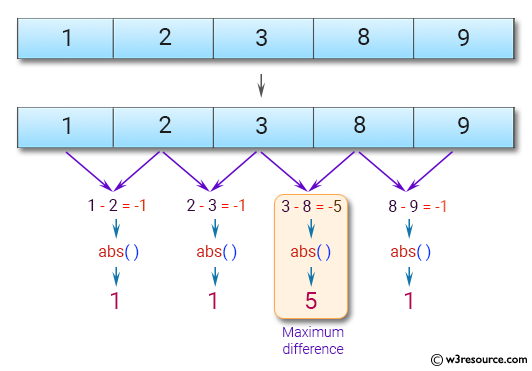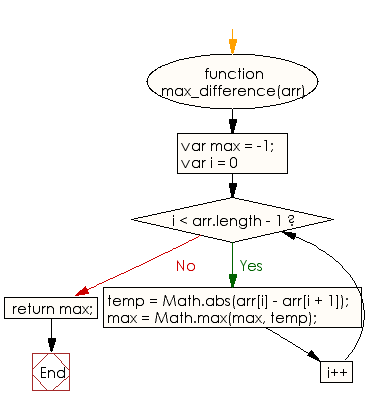# JavaScript: Find the maximum difference between any two adjacent elements of a given array of integers

## JavaScript Basic: Exercise-92 with Solution

Write a JavaScript program to find the maximum difference between any two adjacent elements of a given array of integers.

Pictorial Presentation:Sample Solution:

HTML Code:

``````<!DOCTYPE html>
<html>
<meta charset="utf-8">
<meta name="viewport" content="width=device-width">
<title>Find the maximum difference between any two adjacent elements of a given array of integers.</title>
<body>

</body>
</html>
```
```

JavaScript Code:

``````function max_difference(arr) {
var max = -1;
var temp;
for (var i = 0; i < arr.length - 1; i++)
{
temp = Math.abs(arr[i] - arr[i + 1]);
max = Math.max(max, temp);
}
return max;
}

console.log(max_difference([1, 2, 3, 8, 9]))
console.log(max_difference([1, 2, 3, 18, 9]))
console.log(max_difference([13, 2, 3, 8, 9]))
```
```

Sample Output:

```5
15
11
```

Flowchart:ES6 Version:

``````function max_difference(arr) {
let max = -1;
let temp;
for (let i = 0; i < arr.length - 1; i++)
{
temp = Math.abs(arr[i] - arr[i + 1]);
max = Math.max(max, temp);
}
return max;
}

console.log(max_difference([1, 2, 3, 8, 9]))
console.log(max_difference([1, 2, 3, 18, 9]))
console.log(max_difference([13, 2, 3, 8, 9]))
``````

Live Demo:

See the Pen javascript-basic-exercise-92 by w3resource (@w3resource) on CodePen.

What is the difficulty level of this exercise?

Test your Programming skills with w3resource's quiz.

﻿

## JavaScript: Tips of the Day

Checks if a string is an anagram of another string (case-insensitive, ignores spaces, punctuation and special characters)

Example:

```const isAnagram = (str1, str2) => {
const normalize = str =>
str
.toLowerCase()
.replace(/[^a-z0-9]/gi, '')
.split('')
.sort()
.join('');
return normalize(str1) === normalize(str2);
};
console.log(isAnagram('iceman', 'cinema')); // true
```

Output:

```true
```